# Continuous fraction

The same as continued fraction, i.e. an expression of the form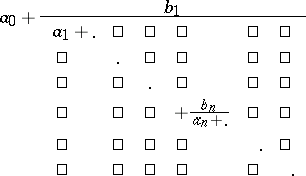where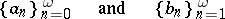are finite or infinite sequences of complex numbers or functions. For continued fractions one uses the notation(*)

As a rule, it is assumed that the sequences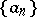and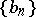are such that for all,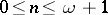,(The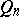are defined recursively, with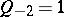,.)

In translations the phrase continuous fraction occurs occasionally instead of continued fraction.

The notation (*) is due to A. Pringsheim, cf. [a1] for other notations.

The number or function obtained by evaluation of the truncationis called the-th convergent of the continued fraction. It equals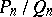where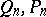satisfy the recursion given above (with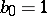) and starting values,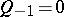;,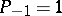. If the convergentstend to a limit as, one says that the continued fraction converges.

Continued fractions play an important role in number theory, in particular in Diophantine approximation, where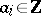,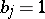, in ergodic theory, again with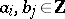, and in numerical mathematics, where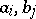are rational functions. Famous examples of explicit continued fractions are those for hypergeometric functions such as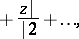whose corresponding's are orthogonal polynomials. In number theory the most famous is the golden ratio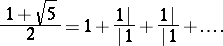Good introductions to continued fractions in number theory are [a1], Chapt. X, [a2], in numerical mathematics [a3], and generally [a4].

How to Cite This Entry:
Continuous fraction. O.A. Ivanova (originator), Encyclopedia of Mathematics. URL: http://encyclopediaofmath.org/index.php?title=Continuous_fraction&oldid=13285
This text originally appeared in Encyclopedia of Mathematics - ISBN 1402006098